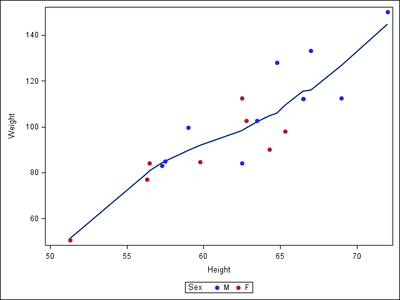## Customizing Proc Loess

I am running proc loess and would like the dots to be different colors based on a variable. The variable values are A, B, or C and I want the dots to be a specific color depending on if they are A, B, or C. I would still like all the data to remain on the same graph. I would also like a legend. I couldn't find the option within proc loess so I was hoping maybe there was a global option or format that could do it.

Thanks

SAS 9.4

``````proc loess data=Veh_All_Ramp1_106 plots(only)=fit;
model speed=distance / smooth=0.5;
run;``````
1 ACCEPTED SOLUTION

Accepted Solutions

## Re: Customizing Proc Loess

The easiest way to accomplish this is to use PROC SGPLOT and overlay a loess curve on top of a scatter plot with the GROUP= option.

``````proc sgplot data=sashelp.cars(obs=100);
scatter x=weight y=mpg_city / group=origin markerattrs=(symbol=CircleFilled);
loess x=weight y=mpg_city / smooth=0.5 nomarkers;
run;``````
2 REPLIES 2

## Re: Customizing Proc Loess

The easiest way to accomplish this is to use PROC SGPLOT and overlay a loess curve on top of a scatter plot with the GROUP= option.

``````proc sgplot data=sashelp.cars(obs=100);
scatter x=weight y=mpg_city / group=origin markerattrs=(symbol=CircleFilled);
loess x=weight y=mpg_city / smooth=0.5 nomarkers;
run;``````PGStats
Opal | Level 21

## Re: Customizing Proc Loess

Here is an example using sashelp.class:

``````proc sgplot data=sashelp.class;
loess x=height y=weight / nomarkers smooth=0.5;
scatter x=height y=weight / group=sex markerattrs=(symbol=circlefilled);
run;``````PG
Discussion stats
• 2 replies
• 797 views
• 2 likes
• 3 in conversation